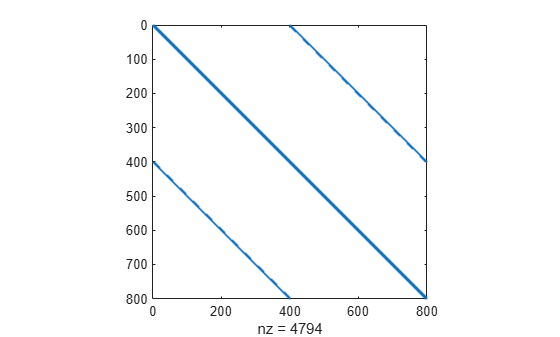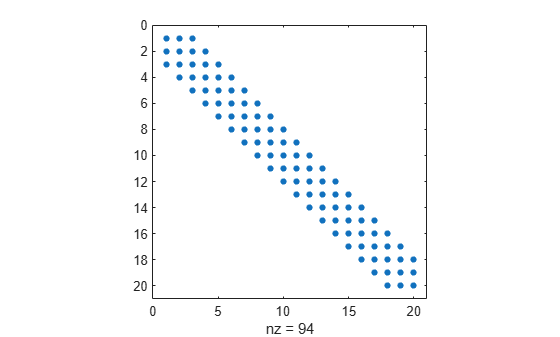# Minimization with Bound Constraints and Banded Preconditioner

This example shows how to solve a nonlinear problem with bounds using the `fmincon` `trust-region-reflective` algorithm. This algorithm provides additional efficiency when the problem is sparse, and has both an analytic gradient and a known structure, such as its Hessian pattern.

### Objective Function with Gradient

For a given $n$ that is a positive multiple of 4, the objective function is

`$f\left(x\right)=1+\sum _{i=1}^{n}{|\left(3-2{x}_{i}\right){x}_{i}-{x}_{i-1}-{x}_{i+1}+1|}^{p}+\sum _{i=1}^{n/2}{|{x}_{i}+{x}_{i+n/2}|}^{p},$`

where $p=7/3$, ${x}_{0}=0$, and ${x}_{n+1}=0$. The `tbroyfg` helper function at the end of this example implements the objective function, including its gradient.

The problem has the bounds $-10\le {x}_{i}\le 10$ for all $i$. Use $n$ = 800.

```n = 800; lb = -10*ones(n,1); ub = -lb;```

### Hessian Pattern

The sparsity pattern of the Hessian matrix is predetermined and stored in the file `tbroyhstr.mat`. The sparsity structure for the Hessian of this problem is banded, as you can see in the following spy plot.

```load tbroyhstr spy(Hstr)```In this plot, the center stripe is itself a five-banded matrix. The following plot shows the matrix more clearly.

`spy(Hstr(1:20,1:20))`### Problem Options

Set options to use the `trust-region-reflective` algorithm. This algorithm requires you to set the `SpecifyObjectiveGradient` option to `true`.

Also, use `optimoptions` to set the `HessPattern` option to `Hstr`. If you do not set this option for such a large problem with an obvious sparsity structure, the problem uses a great amount of memory and computation because `fmincon` attempts to use finite differencing on a full Hessian matrix of 640,000 nonzero entries.

```options = optimoptions('fmincon','SpecifyObjectiveGradient',true,'HessPattern',Hstr,... 'Algorithm','trust-region-reflective');```

### Solve Problem

Set the initial point to –1 for odd indices and +1 for even indices.

```x0 = -ones(n,1); x0(2:2:n) = 1;```

The problem has no linear or nonlinear constraints, so set those parameters to `[]`.

```A = []; b = []; Aeq = []; beq = []; nonlcon = [];```

Call `fmincon` to solve the problem.

```[x,fval,exitflag,output] = ... fmincon(@tbroyfg,x0,A,b,Aeq,beq,lb,ub,nonlcon,options);```
```Local minimum possible. fmincon stopped because the final change in function value relative to its initial value is less than the value of the function tolerance. ```

### Examine Solution and Solution Process

Examine the exit flag, objective function value, first-order optimality measure, and number of solver iterations.

`disp(exitflag);`
``` 3 ```
`disp(fval)`
``` 270.4790 ```
`disp(output.firstorderopt)`
``` 0.0163 ```
`disp(output.iterations)`
``` 7 ```

`fmincon` does not take many iterations to reach a solution. However, the solution has a relatively high first-order optimality measure, which is why the exit flag does not have the preferred value of 1.

### Improve Solution

Try using a five-banded preconditioner instead of the default diagonal preconditioner. Using `optimoptions`, set the `PrecondBandWidth` option to 2 and solve the problem again. (The bandwidth is the number of upper or lower diagonals, not counting the main diagonal.)

```options.PrecondBandWidth = 2; [x2,fval2,exitflag2,output2] = ... fmincon(@tbroyfg,x0,A,b,Aeq,beq,lb,ub,nonlcon,options);```
```Local minimum possible. fmincon stopped because the final change in function value relative to its initial value is less than the value of the function tolerance. ```
`disp(exitflag2);`
``` 3 ```
`disp(fval2)`
``` 270.4790 ```
`disp(output2.firstorderopt)`
``` 7.5340e-05 ```
`disp(output2.iterations)`
``` 9 ```

The exit flag and objective function value do not appear to change. However, the number of iterations increases, and the first-order optimality measure decreases considerably. Compute the difference in objective function value.

`disp(fval2 - fval)`
``` -2.9005e-07 ```

The objective function value decreases by a tiny amount. The solution mainly improves the first-order optimality measure, not the objective function.

### Helper Function

This code creates the `tbroyfg` helper function.

```function [f,grad] = tbroyfg(x,dummy) %TBROYFG Objective function and its gradients for nonlinear minimization % with bound constraints and banded preconditioner. % Documentation example. % Copyright 1990-2008 The MathWorks, Inc. n = length(x); % n should be a multiple of 4 p = 7/3; y=zeros(n,1); i = 2:(n-1); y(i) = abs((3-2*x(i)).*x(i) - x(i-1) - x(i+1)+1).^p; y(n) = abs((3-2*x(n)).*x(n) - x(n-1)+1).^p; y(1) = abs((3-2*x(1)).*x(1) - x(2)+1).^p; j = 1:(n/2); z = zeros(length(j),1); z(j) = abs(x(j) + x(j+n/2)).^p; f = 1 + sum(y) + sum(z); % % Evaluate the gradient. if nargout > 1 g = zeros(n,1); t = zeros(n,1); i = 2:(n-1); t(i) = (3-2*x(i)).*x(i) - x(i-1) - x(i+1) + 1; g(i) = p*abs(t(i)).^(p-1).*sign(t(i)).*(3-4*x(i)); g(i-1) = g(i-1) - p*abs(t(i)).^(p-1).*sign(t(i)); g(i+1) = g(i+1) - p*abs(t(i)).^(p-1).*sign(t(i)); tt = (3-2*x(n)).*x(n) - x(n-1) + 1; g(n) = g(n) + p*abs(tt).^(p-1).*sign(tt).*(3-4*x(n)); g(n-1) = g(n-1) - p*abs(tt).^(p-1).*sign(tt); tt = (3-2*x(1)).*x(1)-x(2)+1; g(1) = g(1) + p*abs(tt).^(p-1).*sign(tt).*(3-4*x(1)); g(2) = g(2) - p*abs(tt).^(p-1).*sign(tt); j = 1:(n/2); t(j) = x(j) + x(j+n/2); g(j) = g(j) + p*abs(t(j)).^(p-1).*sign(t(j)); jj = j + (n/2); g(jj) = g(jj) + p*abs(t(j)).^(p-1).*sign(t(j)); grad = g; end end```